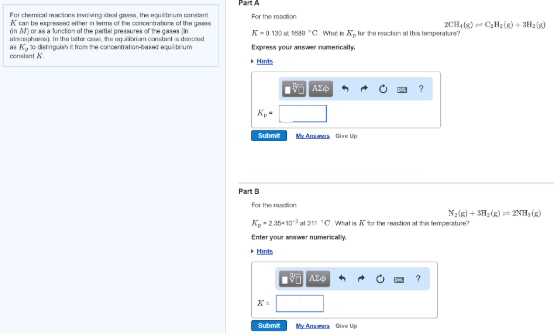# Problem: For chemical reactions involving ideal gases, the equilibrium constant K can be expressed either in terms of the concentrations of the gases (in M) or as a function of the partial pressures of the gases (in atmospheres). In the latter case, the equilibrium constant is denoted as Kp to distinguish it from the concentration-based equilibrium constant K. For the reaction 2CH4 (g) ⇌ C2H2(g) + 3H2(g)K = 0.130 at 1689°C. What is Kp for the reaction at this temperature?For the reaction N2(g) + 3H2(g) ⇌ 2NH3(g)Kp = 2.35 x 10-3 at 311°C  K = 0.130 at 1689 °C. What is Kp for the reaction at this temperature?

###### FREE Expert Solution
94% (471 ratings)###### Problem Details

For chemical reactions involving ideal gases, the equilibrium constant K can be expressed either in terms of the concentrations of the gases (in M) or as a function of the partial pressures of the gases (in atmospheres). In the latter case, the equilibrium constant is denoted as Kp to distinguish it from the concentration-based equilibrium constant K.

For the reaction 2CH4 (g) ⇌ C2H2(g) + 3H2(g)

K = 0.130 at 1689°C. What is Kp for the reaction at this temperature?

For the reaction N2(g) + 3H2(g) ⇌ 2NH3(g)

Kp = 2.35 x 10-3 at 311°C  K = 0.130 at 1689 °C. What is Kp for the reaction at this temperature?